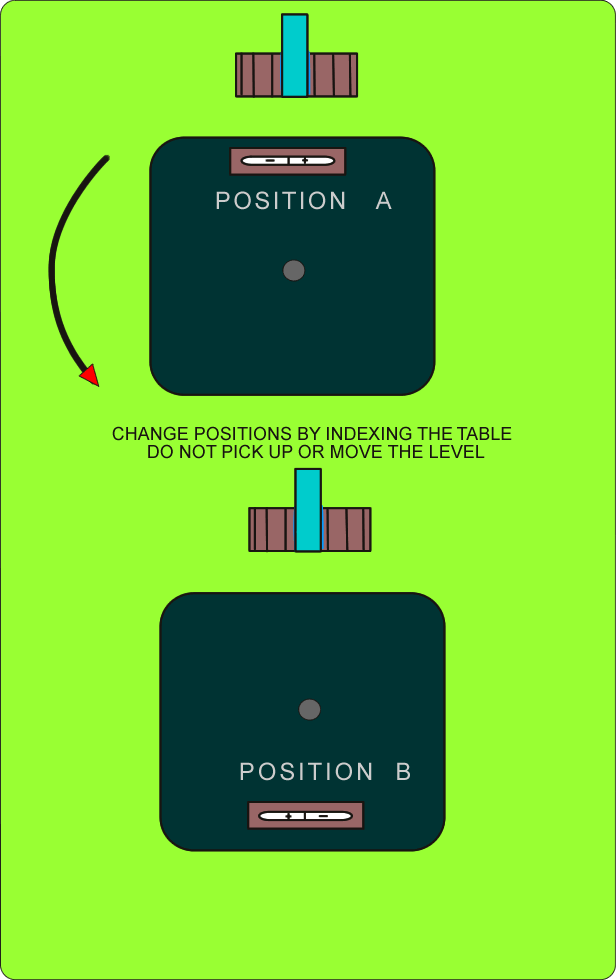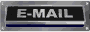` `

To convert arc sec. into inches

1 second =.000005" per 1"
1 second = .000048" per 10"
1 second = .000058" per 12"
```
The formula works for both front to back and left to right```

Tip

Use the side of the table were the errors cancel each other to your advantage until you can get it fixedCALCULATIONS FOR ROTARY TABLE AND SADDLE WEAR ISOLATION You would use it to determine which part of your machine needs service You will need a precision level and a calculator Step one Level the machines bed ways for datum . We will assume the machines bed ways are level with the horizon in the following procedures. Step Two Take the readings and plug them into the formula Be especially careful of the signs +/- ``` Formula (Position A Reading) - (Position B Reading) /2 = Saddle Error ```   (Position A reading) - (Saddle Error) = Rotary Table Error ``` ``` ``` ``` ``` ``` ``` ``` ``` ``` ``` ``` ``` ```